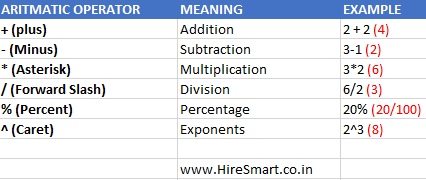## Calculation Operators In Excel Formulas & Their Priority Order For Use

As explained on the previous page, that one of the parts or elements commonly used in an Excel formula is the excel operator.

What is an excel operator? What are the types of operators in Excel? What is the order of using operators in Excel formulas? These questions will be answered in this excel tutorial.

## Understanding Excel Operators

Excel operators are symbols or signs that have a specific function and are used to perform data processing or calculations.

Operators in Excel determine the type of calculation we want to run on the elements of an Excel formula such as addition, subtraction, multiplication, or division and so on.

In general, the use of excel operators follows the order of general mathematical calculation rules. Suppose the multiplication and division operators take precedence over addition and subtraction operators. However, we can change this order using the "(...)" brackets.

There aren't just 7 or 8 math operators only that applies in Excel with the order level from high to low as is often known as follows:

1. Rank (^)
2. Percent (%)
3. Multiplication (*)
4. Division (/)
6. Subtraction (-)
7. String concatenation of text (&)
8. Grouping or Grouping with brackets "(...)"

In Excel there are four types of calculation operators, namely arithmetic operators , comparison operators , text operators and reference operators . So, what operators are included in the four types of excel operators?

##Excel Arithmetic Operators or math operators are used to perform basic math operations, such as addition, subtraction, multiplication, or division. As well as to combine numbers and or generate numeric values.

Arithmetic Operators in Microsoft Excel

OPERATOR MEANING
- (Minus / Less) Math operators for subtraction
* (Asterisk / star) Mathematical operators used for multiplication functions
/ (Slash) Mathematical operators that function to divide
% (Percent / Percentage) Mathematical operators that function to express numbers in percent (per hundred)
^ (Caret / Insert) Math operators for ranks

The order of operator levels will be discussed at the end of this excel tutorial.

##When two values ​​are compared using this excel comparison operator, the result is a logical value TRUE or FALSE.

This is kind of comparison operator that will often we need while using the logic functions in compiling excel formulas such as the IF function, an OR function , AND function and function NOT .

Comparison Operators in Microsoft Excel

OPERATOR MEANING
= Together with ...
> More than ...
< Less than ...
> = Greater than or Equal to ...
<= Less than or equal to ...
<> Not equal to ...

## Text Operators in Excel

Use an ampersand (&) to concatenate one or more text strings to produce a single text. In addition to using ampersands, Microsoft Excel also provides a special function for combining 2 or more texts by using the CONCANTENATE function.

## Excel Reference Operators

What are reference operators?

Reference operators are generally used to create a cell reference or range in Excel.

Which includes excel reference operators, among others:

• Colon ":" indicates the range of cells or cell range. Example B5: B9, shows the cell ranges B5, B6, B7, B8 and B9.
• The "" space indicates a slice of a cell range. Often the use of this operator causes an error.

The Ranking Order of Operators in Microsoft Excel

What is the sequence of calculations in Microsoft Excel formulas?

When Excel performs calculations on a formula, the order of the calculations is very important. Because it can affect the value generated by an excel formula that you use in a cell.

As in mathematical operations where the calculation of multiplication and division takes precedence over addition and subtraction. Excel also performs calculations from left to right in order of a certain level or priority to the excel operator used.

Every Excel formula always starts with an equal sign (=). All characters after this sign are part of an excel formula, whether it is a number, text, reference, operator or excel function.

Excel calculates the formula from left to right, according to a specific order for each operator in the formula. The following is the priority or work order of the operators in Excel.

The calculation process in Excel will be carried out according to the degree order of the excel operator, namely:

1) Reference Operators.

• Colon (:)
• Space ()
• Comma (,)

2) Negativity. For example (-1)

3) Percent (%)

4) Power (^)

5) Multiplication and division (* and /)

6) Addition and subtraction (+ and -)

7) Text operator (&)

8) Comparison operator

• = (Same as)
• > (More than)
• <(Less than)
• > = (More than or equal to)
• <= (Less than or equal to)
• <> (Not the same as)

As in general mathematical calculations, to change the order of evaluation of calculations you can use parentheses to mark the part of the formula that must be calculated first.

This is a brief explanation of the calculation operators in Excel. If something is not understood, do not hesitate to leave a message.

Before moving on, don't forget to bookmark and share this excel tutorial article with the buttons provided below. Greetings Excel Class.